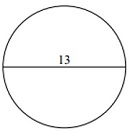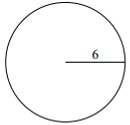# Area of a Circle

The area of a circle is the extent of interior of a circle.

The area of a circle is the number of square units inside that circle. If each square in the circle below has an area of 1 cm2, we can count the total number of squares to get the area of this circle. Thus, if there were a total of 28.24 squares, the area of this circle would be 28.24 cm2However, it is easier to use one of the following formulas −

Formula for Area of a Circle

• Area of a circle is given by the formula A = πr2 where π = 3.14 and r is the radius of the circle.

• Area of a circle is given by the formula A = πd2/4 where π = 3.14 and d is the diameter of the circle.

Find the area of following circle. Use π = 3.14### Solution

Step 1:

Given diameter = 13; radius r = 13/2 = 6.5 units

Step 2:

Area of Circle = πr2 = (3.14)(6.5)(6.5) = 132.67 square units

Find the area of following circle. Use π = 3.14### Solution

Step 1:

Given radius r = 6 units

Step 2:

Area of Circle = πr2 = (3.14)(6)(6) = 113.04 square units